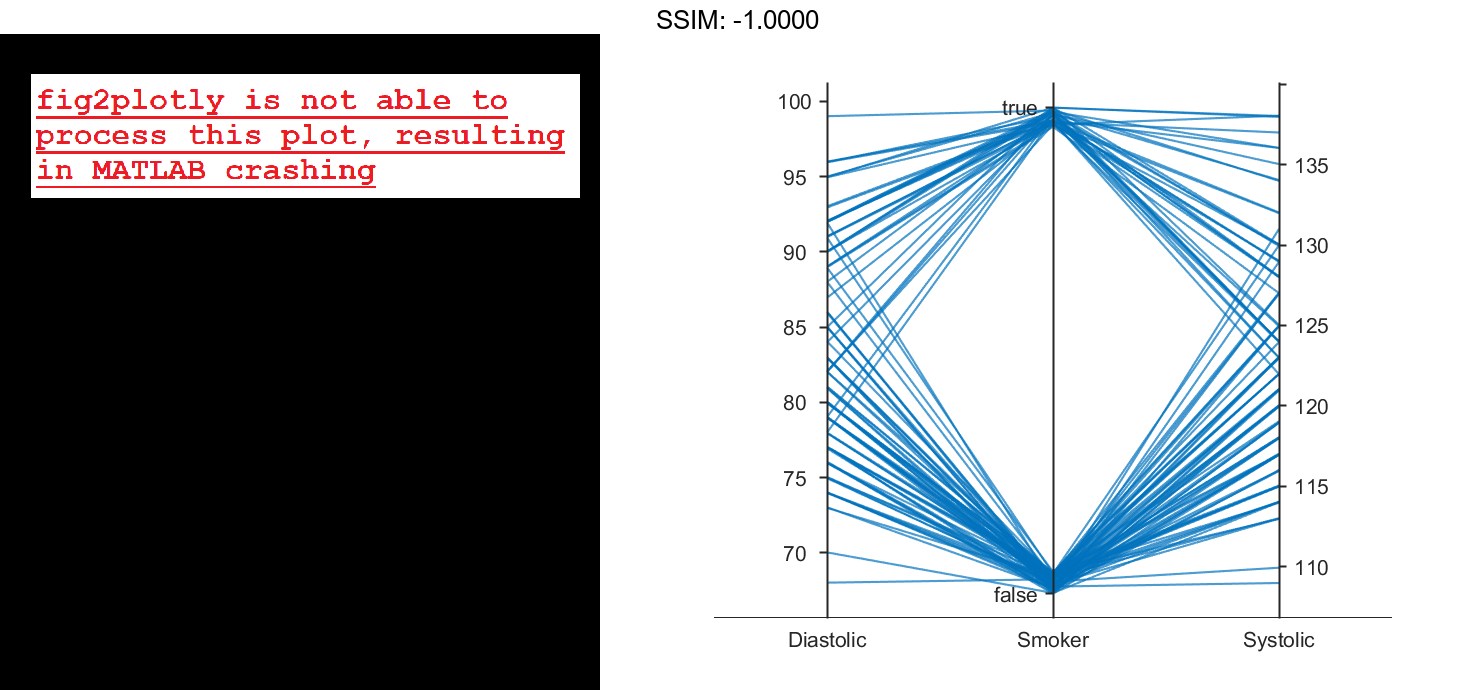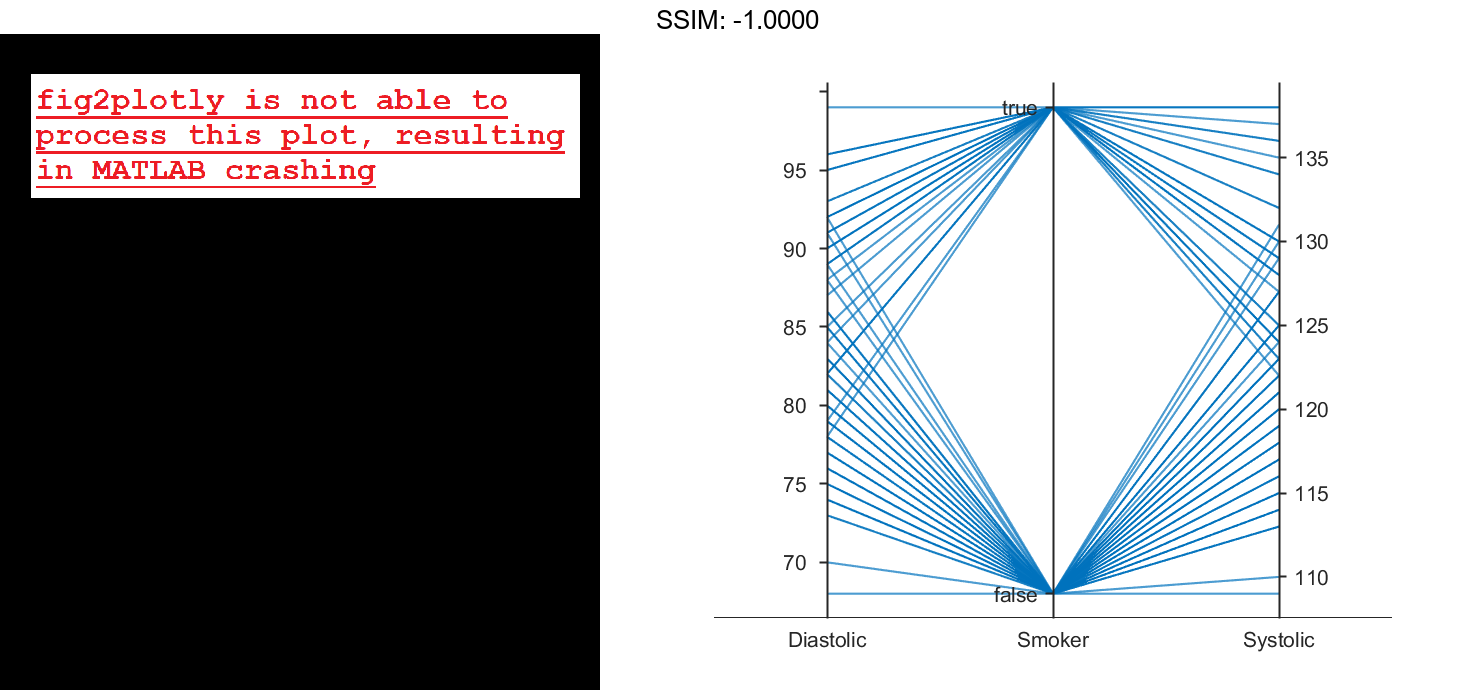# Parallel Coordinates Plot in MATLAB®

How to make Parallel Coordinates Plot in MATLAB® with Plotly.

## Parallel Coordinates Plot with Tabular Data

Create a parallel coordinates plot from a table of medical patient data.

Load the patients data set, and create a table from a subset of the variables loaded into the workspace. Create a parallel coordinates plot using the table. The lines in the plot correspond to individual patients. Use the plot to observe trends in the data. For example, the plot indicates that smokers tend to have higher blood pressure values (both diastolic and systolic).

load patients
tbl = table(Diastolic,Smoker,Systolic);
p = parallelplot(tbl)

fig2plotly()p =
ParallelCoordinatesPlot with properties:

SourceTable: [100x3 table]
CoordinateVariables: {'Diastolic'  'Smoker'  'Systolic'}
GroupVariable: ''

Show all properties



By default, the software randomly jitters plot lines so that they are unlikely to overlap perfectly along coordinate rulers. This jittering is particularly helpful for visualizing categorical data because it enables you to distinguish between plot lines more easily. For example, observe the plot lines along the Smoker coordinate ruler; the plot lines are not flush with either the true or false tick marks.

To disable the default jittering, set the Jitter property to 0.

p.Jitter = 0;

fig2plotly()## Specify Coordinate and Group Variables

Create a parallel coordinates plot from a table of tsunami data. Specify the table variables to display and their order, and group the lines in the plot according to one of the variables.

Read the tsunami data into the workspace as a table.

tsunamis = readtable('tsunamis.xlsx');


Create a parallel coordinates plot using a subset of the variables in the table. First, increase the figure window size to prevent overcrowding in the plot. Then, to specify the variables and their order, use the 'CoordinateVariables' name-value pair argument. To group occurrences according to their validity, set the 'GroupVariable' name-value pair argument to 'Validity'. The lines in the plot correspond to individual tsunami occurrences. The plot indicates that most of the occurrences in the data set that have a Validity value are considered definite tsunamis.

figure('Units','normalized','Position',[0.3 0.3 0.45 0.4])
coordvars = {'Year','Validity','Cause','Country'};
p = parallelplot(tsunamis,'CoordinateVariables',coordvars,'GroupVariable','Validity');

fig2plotly()## Parallel Coordinates Plot with Binned Data

Create a parallel coordinates plot from a matrix containing medical patient data. Bin the values in one of the columns in the matrix, and group the lines in the plot using the binned values.

Load the patients data set, and create a matrix from the Age, Height, and Weight values. Create a parallel coordinates plot using the matrix data. Label the coordinate variables in the plot. The lines in the plot correspond to individual patients.

load patients
X = [Age Height Weight];
p = parallelplot(X)

fig2plotly()

p =
ParallelCoordinatesPlot with properties:

Data: [100x3 double]
CoordinateData: [1 2 3]
GroupData: []

Show all properties


p.CoordinateTickLabels = {'Age (years)','Height (inches)','Weight (pounds)'};

fig2plotly()